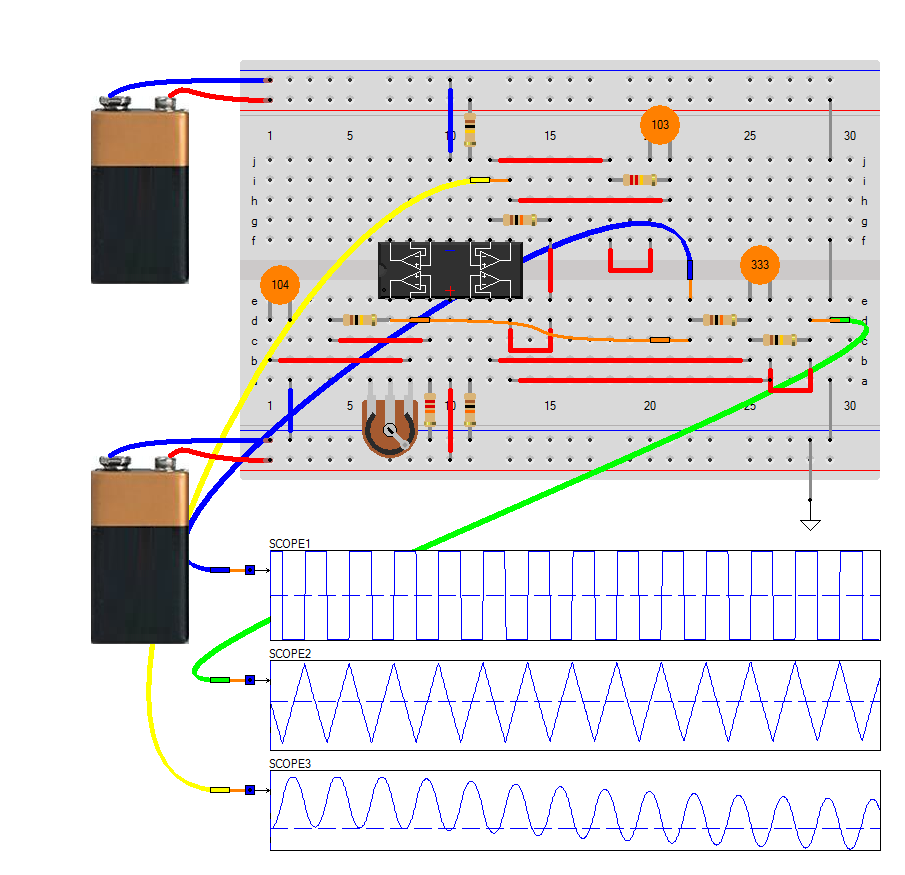## Signal Generator

A function generator is a electronic device that can produce a variety of different waveforms. The one we will build can output square, triangle, and sine waveforms. Like standard function generators, the circuit allows for frequency adjustment by means of a potentiometer.

The circuit works on the principle of just using op amps. The LM324 is a quad op amp, meaning it's composed of 4 independent op amps. In this circuit, the first op amp produces a square wave. After that, the circuit uses 2 integrator circuits to convert the square wave into triangle and sine wave signals.

The function generator circuit we will build with an LM324 op amp chip is shown below.This circuit is pretty basic. The first op amp produces a square wave. We feed this square wave into an integrator circuit, which outputs a triangle wave. We then feed this triangle wave into a second integrator circuit, which outputs a sine wave.

The first opamp produces a square waveform. The 100KΩ potentiometer allows us to vary the frequency to the circuit. And it's the way of adjusting the frequency of the output signal.

After the first op amp, we have a square waveform. What follows next is an integrator circuit. When you feed a square waveform into an integrator circuit, the output is a triangle waveform.

After the second op amp, we now have a triangle waveform, as our second waveform. We then feed this triangle waveform into another integrator circuit. When you feed a triangle waveform into an integrator circuit, the output is a sine waveform. However all depends heavily on the choice of the integrator constants made up by the R and C in the feedback of each opamp.